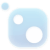# Top 20 NuGet distance Packages

PhoneticMatching C# project.
.NET object to handle distance.
Calculate distance between two string by levenshtein distance algorithm
A small evaluation tool to calculate the jaccard similarity index and distance. It also includes mean jaccard index and distance which considers the order of the elements as well.
Common String, SecureString, and Collection Extension Methods which include: ToSecureString, ToInsecureString, EqualsIgnoreCase, ContainsIgnoreCase, Before, After, FuzzyEquals, IsNullOrEmpty, and IsNullOrWhiteSpace. Additionally there are helper methods and classes that simplify working with ...
A C# implementation of the Levenshtein string distance, using lazy evaluation of its diagonals. Time Complexity: O( |a| * dist(A,B) ) Supports thresholding to skip calculations when distance is greater than the threshold https://www.codeinthenorth.com## Levenshtein Distance And String Similarity Ratio

this package is based on Levenshtein Distance Algorithm: eg. first string ="abc rs t" secind string ="acb ts t" levenshtein distance =3 similarity ratio = 0.625 it is useful to understand how much both input strings are similar.